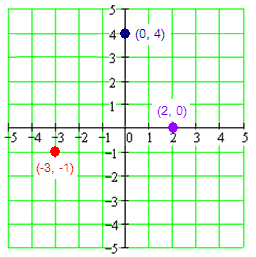# The Rectangular Coordinate System and Point Plotting

The rectangular coordinate system is also known as the Cartesiancoordinate system after Rene Descartes.

The rectangular coordinate system is based on a grid, and every point on the plane can be identified by unique x and y coordinates, just as any point on the Earth can be identified by giving its latitude and longitude.Axes

Locations on the grid are measured relative to a fixed point, called the origin, and are measured according to the distance along a pair of axes.

The x and y axes are just like the number line, with positive distances to the right and negative to the left in the case of the x axis, and positive distances measured upwards and negative down for the y axis.

Any displacement away from the origin can be constructed by moving a specified distance in the x direction and then another distance in the ydirection.

Think of it as if you were giving directions to someone by saying something like "go three blocks East and then 2 blocks North."

Coordinates, Graphing Points

We specify the location of a point by first giving its x coordinate (the left or right displacement from the origin), and then the y coordinate (the up or down displacement from the origin). Thus, every point on the plane can be identified by a pair of numbers (x,y), called its coordinates.Sometimes we just want to know what general part of the graph we are talking about. The axes naturally divide the plane up into quarters. We call these quadrants, and number them from one to four. Notice that the numbering begins in the upper right quadrant and continues around in the counter–clockwise direction. Notice also that each quadrant can be identified by the unique combination of positive and negative signs for the coordinates of a point in that quadrant.The rectangular coordinate system, also called the Cartesian coordinate system or the x–y coordinate system is shown above.

Notice that the rectangular coordinate system consists of 4 quadrants, a horizontal axis, a vertical axis, and the origin. The horizontal axis is usually called the x–axis, and the vertical axis is usually called the y–axis. The origin is the point where the two axes cross.

Example:

Plot each of the following points. A(–3, –1), B(0, 4), and C(2, 0).

Solution:

A(–3, –1): From the origin, go to the left 3 units then down 1 unit. Then plot the point.

B(0, 4) From the origin, go straight up 4 units.

C(2, 0) From the origin, go 2 units to the right and plot the point.In which quadrant is the point (-2, 4) located?
Find the distance from (-6, 1) to (-3, 5).
In the xy-coordinate plane, in which quadrant is xy < 0?

a) Q II

b) Q III

c) Q IV

d) Q II and Q IV